# RD Sharma Solutions for Class 6 Maths Chapter 20: Mensuration Exercise 20.2

The students can make use of solutions designed by subject matter experts in order to understand the concepts in an effective way. This exercise mainly helps students get to know about the important formulas which are used to find the perimeter of geometrical structures like rectangle, square and equilateral triangle. These solutions help students self analyse their knowledge about the concepts which are covered in each exercise. Students who aspire to perform well in the exams can use RD Sharma Solutions Class 6 Maths Chapter 20 Mensuration Exercise 20.2 PDF from the links provided below.

## RD Sharma Solutions for Class 6 Maths Chapter 20: Mensuration Exercise 20.2 Download PDF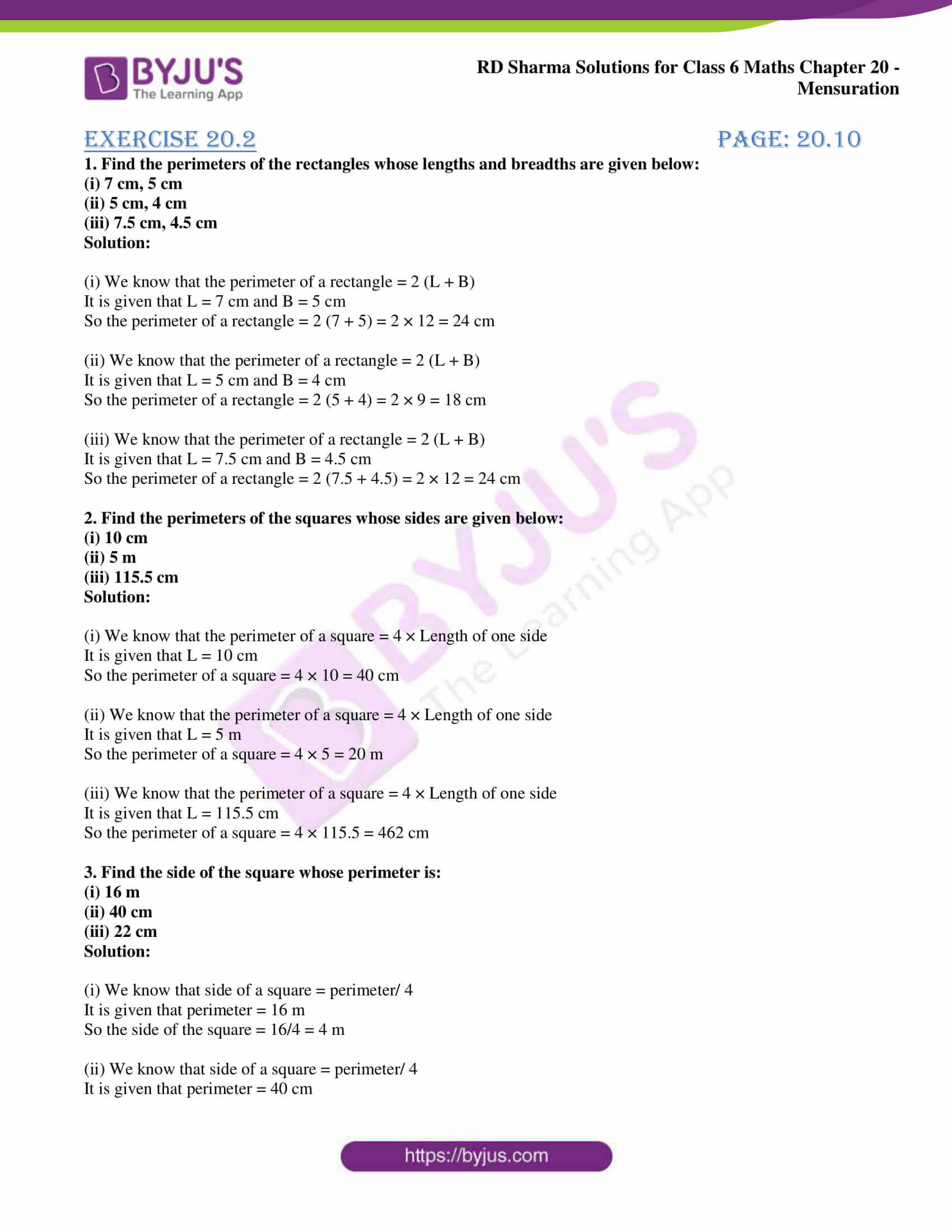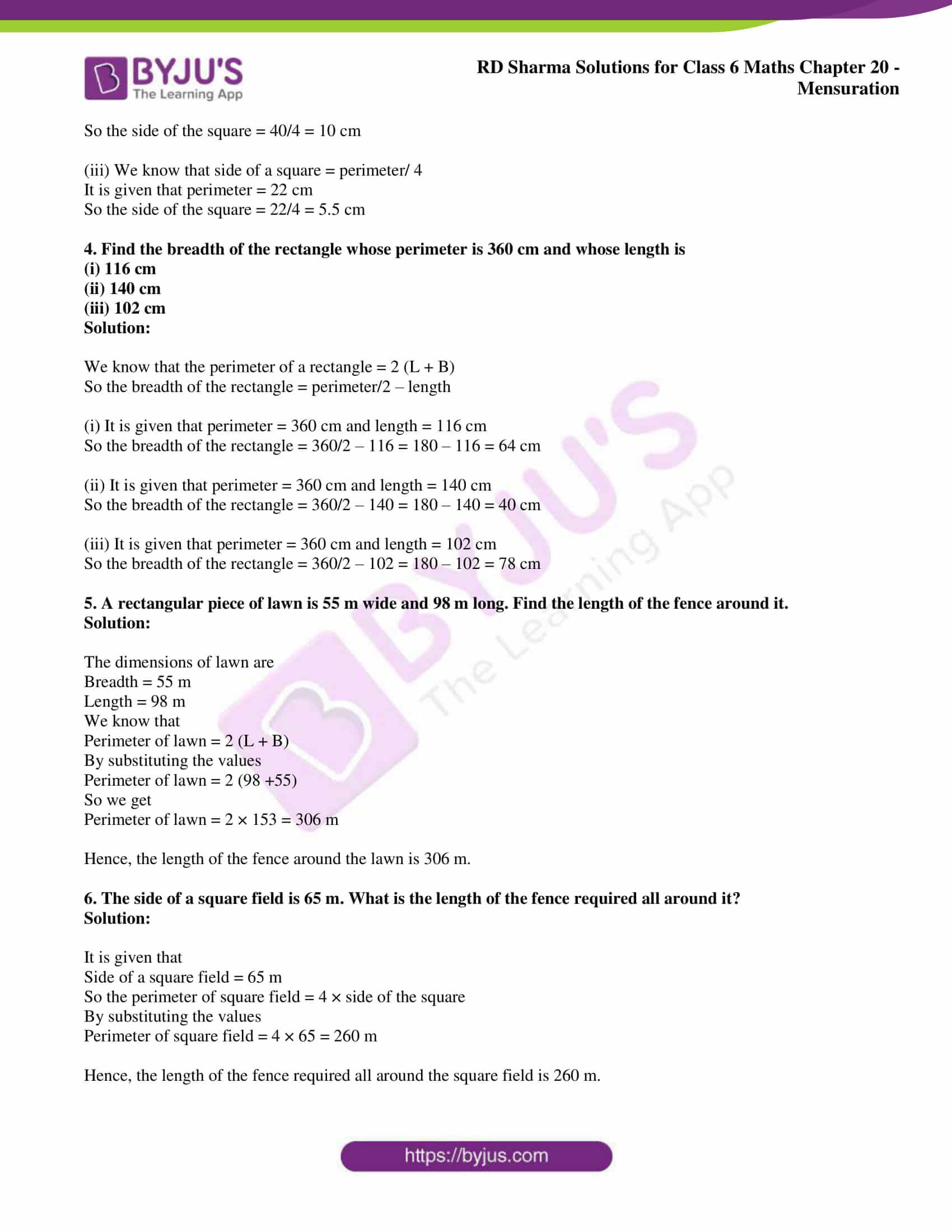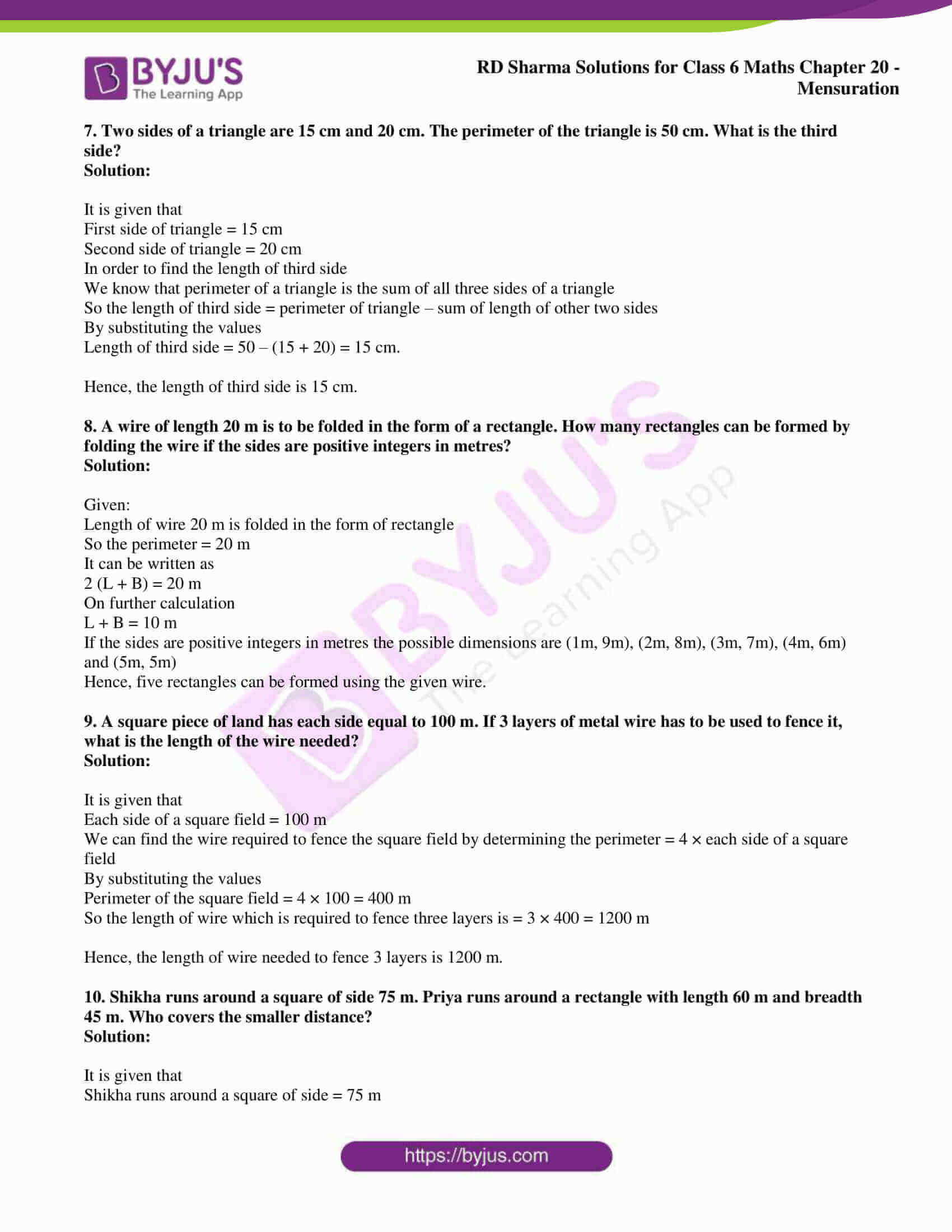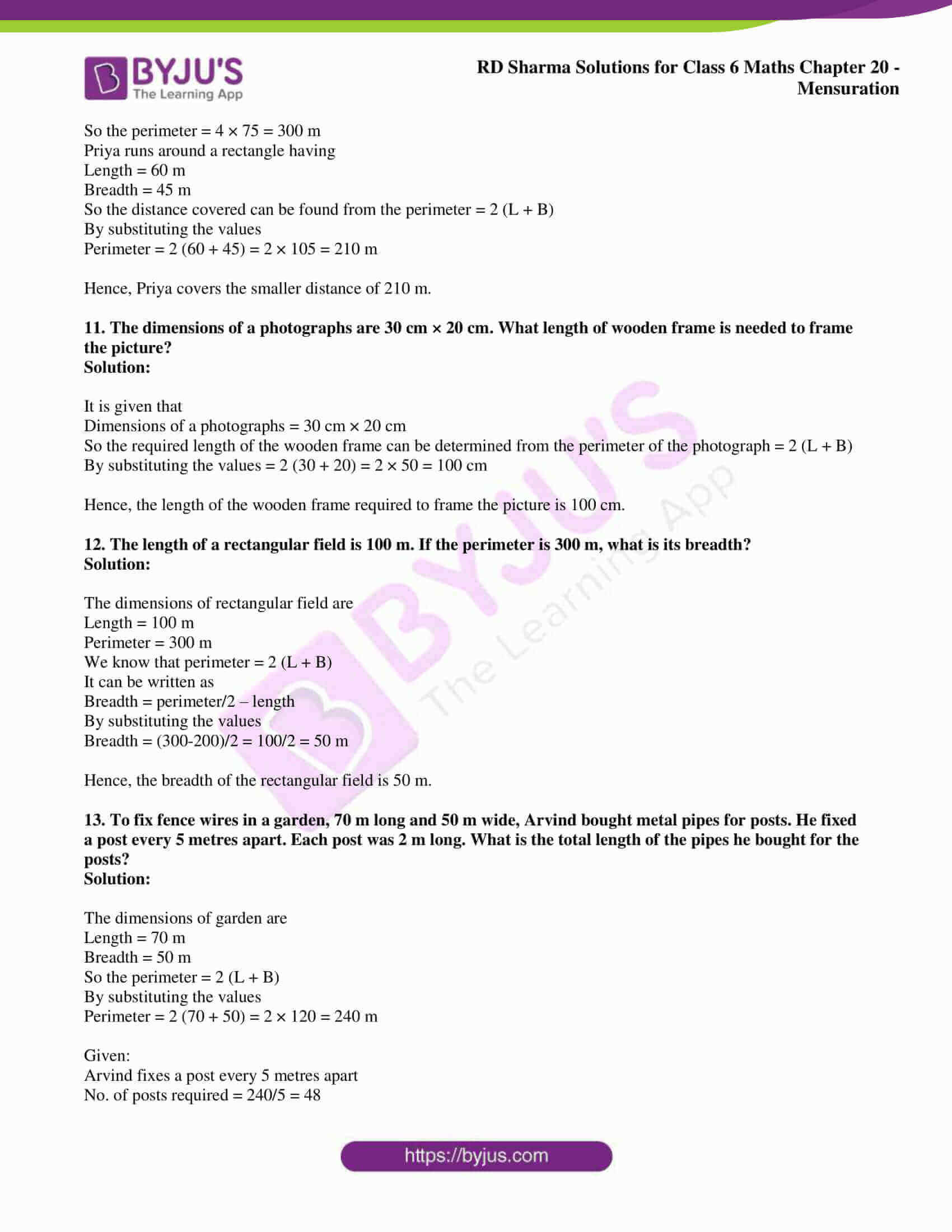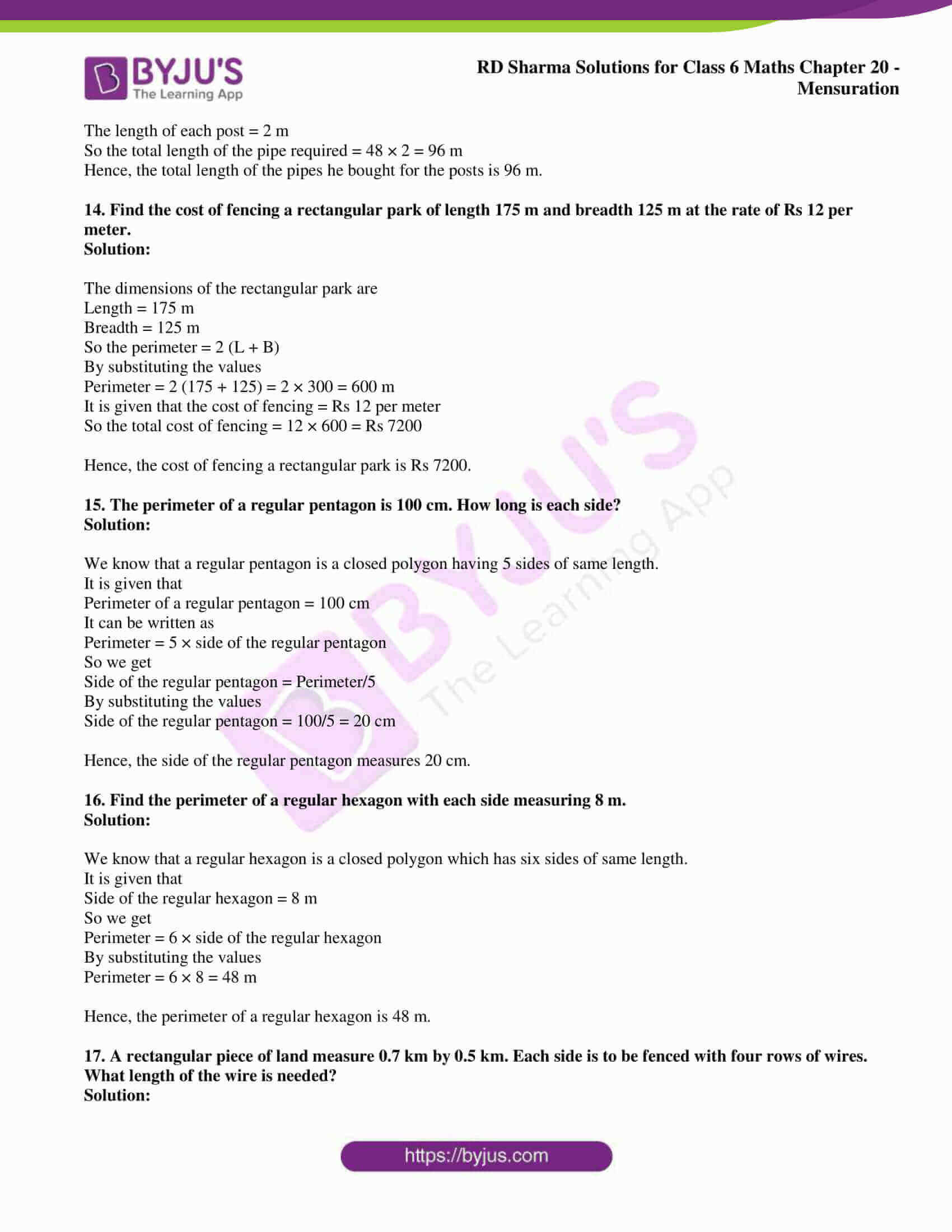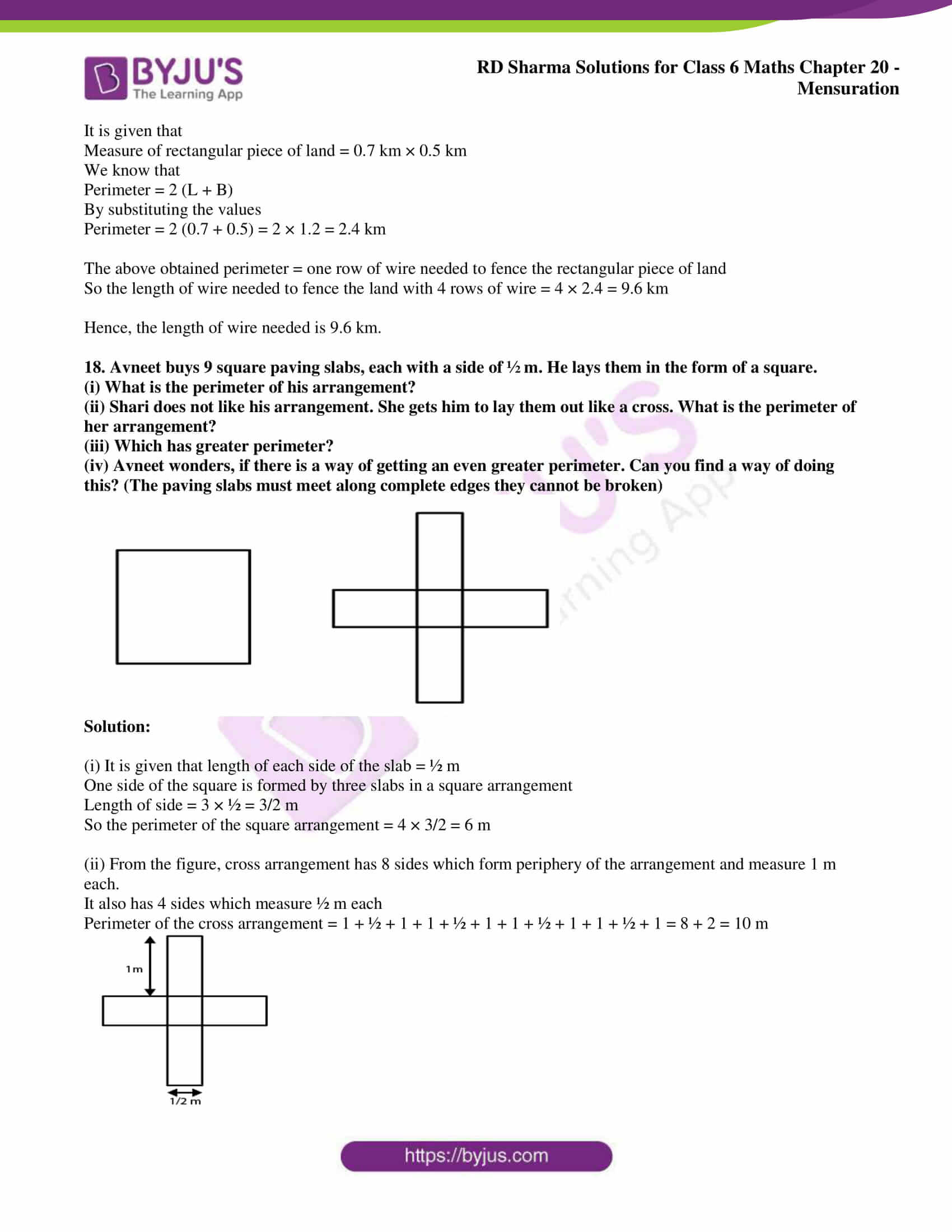### Exercise 20.2 page: 20.10

1. Find the perimeters of the rectangles whose lengths and breadths are given below:

(i) 7 cm, 5 cm

(ii) 5 cm, 4 cm

(iii) 7.5 cm, 4.5 cm

Solution:

(i) We know that the perimeter of a rectangle = 2 (L + B)

It is given that L = 7 cm and B = 5 cm

So the perimeter of a rectangle = 2 (7 + 5) = 2 × 12 = 24 cm

(ii) We know that the perimeter of a rectangle = 2 (L + B)

It is given that L = 5 cm and B = 4 cm

So the perimeter of a rectangle = 2 (5 + 4) = 2 × 9 = 18 cm

(iii) We know that the perimeter of a rectangle = 2 (L + B)

It is given that L = 7.5 cm and B = 4.5 cm

So the perimeter of a rectangle = 2 (7.5 + 4.5) = 2 × 12 = 24 cm

2. Find the perimeters of the squares whose sides are given below:

(i) 10 cm

(ii) 5 m

(iii) 115.5 cm

Solution:

(i) We know that the perimeter of a square = 4 × Length of one side

It is given that L = 10 cm

So the perimeter of a square = 4 × 10 = 40 cm

(ii) We know that the perimeter of a square = 4 × Length of one side

It is given that L = 5 m

So the perimeter of a square = 4 × 5 = 20 m

(iii) We know that the perimeter of a square = 4 × Length of one side

It is given that L = 115.5 cm

So the perimeter of a square = 4 × 115.5 = 462 cm

3. Find the side of the square whose perimeter is:

(i) 16 m

(ii) 40 cm

(iii) 22 cm

Solution:

(i) We know that side of a square = perimeter/ 4

It is given that perimeter = 16 m

So the side of the square = 16/4 = 4 m

(ii) We know that side of a square = perimeter/ 4

It is given that perimeter = 40 cm

So the side of the square = 40/4 = 10 cm

(iii) We know that side of a square = perimeter/ 4

It is given that perimeter = 22 cm

So the side of the square = 22/4 = 5.5 cm

4. Find the breadth of the rectangle whose perimeter is 360 cm and whose length is

(i) 116 cm

(ii) 140 cm

(iii) 102 cm

Solution:

We know that the perimeter of a rectangle = 2 (L + B)

So the breadth of the rectangle = perimeter/2 – length

(i) It is given that perimeter = 360 cm and length = 116 cm

So the breadth of the rectangle = 360/2 – 116 = 180 – 116 = 64 cm

(ii) It is given that perimeter = 360 cm and length = 140 cm

So the breadth of the rectangle = 360/2 – 140 = 180 – 140 = 40 cm

(iii) It is given that perimeter = 360 cm and length = 102 cm

So the breadth of the rectangle = 360/2 – 102 = 180 – 102 = 78 cm

5. A rectangular piece of lawn is 55 m wide and 98 m long. Find the length of the fence around it.

Solution:

The dimensions of lawn are

Length = 98 m

We know that

Perimeter of lawn = 2 (L + B)

By substituting the values

Perimeter of lawn = 2 (98 +55)

So we get

Perimeter of lawn = 2 × 153 = 306 m

Hence, the length of the fence around the lawn is 306 m.

6. The side of a square field is 65 m. What is the length of the fence required all around it?

Solution:

It is given that

Side of a square field = 65 m

So the perimeter of square field = 4 × side of the square

By substituting the values

Perimeter of square field = 4 × 65 = 260 m

Hence, the length of the fence required all around the square field is 260 m.

7. Two sides of a triangle are 15 cm and 20 cm. The perimeter of the triangle is 50 cm. What is the third side?

Solution:

It is given that

First side of triangle = 15 cm

Second side of triangle = 20 cm

In order to find the length of third side

We know that perimeter of a triangle is the sum of all three sides of a triangle

So the length of third side = perimeter of triangle – sum of length of other two sides

By substituting the values

Length of third side = 50 – (15 + 20) = 15 cm.

Hence, the length of third side is 15 cm.

8. A wire of length 20 m is to be folded in the form of a rectangle. How many rectangles can be formed by folding the wire if the sides are positive integers in metres?

Solution:

Given:

Length of wire 20 m is folded in the form of rectangle

So the perimeter = 20 m

It can be written as

2 (L + B) = 20 m

On further calculation

L + B = 10 m

If the sides are positive integers in metres the possible dimensions are (1m, 9m), (2m, 8m), (3m, 7m), (4m, 6m) and (5m, 5m)

Hence, five rectangles can be formed using the given wire.

9. A square piece of land has each side equal to 100 m. If 3 layers of metal wire has to be used to fence it, what is the length of the wire needed?

Solution:

It is given that

Each side of a square field = 100 m

We can find the wire required to fence the square field by determining the perimeter = 4 × each side of a square field

By substituting the values

Perimeter of the square field = 4 × 100 = 400 m

So the length of wire which is required to fence three layers is = 3 × 400 = 1200 m

Hence, the length of wire needed to fence 3 layers is 1200 m.

10. Shikha runs around a square of side 75 m. Priya runs around a rectangle with length 60 m and breadth 45 m. Who covers the smaller distance?

Solution:

It is given that

Shikha runs around a square of side = 75 m

So the perimeter = 4 × 75 = 300 m

Priya runs around a rectangle having

Length = 60 m

So the distance covered can be found from the perimeter = 2 (L + B)

By substituting the values

Perimeter = 2 (60 + 45) = 2 × 105 = 210 m

Hence, Priya covers the smaller distance of 210 m.

11. The dimensions of a photographs are 30 cm × 20 cm. What length of wooden frame is needed to frame the picture?

Solution:

It is given that

Dimensions of a photographs = 30 cm × 20 cm

So the required length of the wooden frame can be determined from the perimeter of the photograph = 2 (L + B)

By substituting the values = 2 (30 + 20) = 2 × 50 = 100 cm

Hence, the length of the wooden frame required to frame the picture is 100 cm.

12. The length of a rectangular field is 100 m. If the perimeter is 300 m, what is its breadth?

Solution:

The dimensions of rectangular field are

Length = 100 m

Perimeter = 300 m

We know that perimeter = 2 (L + B)

It can be written as

By substituting the values

Breadth = (300-200)/2 = 100/2 = 50 m

Hence, the breadth of the rectangular field is 50 m.

13. To fix fence wires in a garden, 70 m long and 50 m wide, Arvind bought metal pipes for posts. He fixed a post every 5 metres apart. Each post was 2 m long. What is the total length of the pipes he bought for the posts?

Solution:

The dimensions of garden are

Length = 70 m

So the perimeter = 2 (L + B)

By substituting the values

Perimeter = 2 (70 + 50) = 2 × 120 = 240 m

Given:

Arvind fixes a post every 5 metres apart

No. of posts required = 240/5 = 48

The length of each post = 2 m

So the total length of the pipe required = 48 × 2 = 96 m

Hence, the total length of the pipes he bought for the posts is 96 m.

14. Find the cost of fencing a rectangular park of length 175 m and breadth 125 m at the rate of Rs 12 per meter.

Solution:

The dimensions of the rectangular park are

Length = 175 m

So the perimeter = 2 (L + B)

By substituting the values

Perimeter = 2 (175 + 125) = 2 × 300 = 600 m

It is given that the cost of fencing = Rs 12 per meter

So the total cost of fencing = 12 × 600 = Rs 7200

Hence, the cost of fencing a rectangular park is Rs 7200.

15. The perimeter of a regular pentagon is 100 cm. How long is each side?

Solution:

We know that a regular pentagon is a closed polygon having 5 sides of same length.

It is given that

Perimeter of a regular pentagon = 100 cm

It can be written as

Perimeter = 5 × side of the regular pentagon

So we get

Side of the regular pentagon = Perimeter/5

By substituting the values

Side of the regular pentagon = 100/5 = 20 cm

Hence, the side of the regular pentagon measures 20 cm.

16. Find the perimeter of a regular hexagon with each side measuring 8 m.

Solution:

We know that a regular hexagon is a closed polygon which has six sides of same length.

It is given that

Side of the regular hexagon = 8 m

So we get

Perimeter = 6 × side of the regular hexagon

By substituting the values

Perimeter = 6 × 8 = 48 m

Hence, the perimeter of a regular hexagon is 48 m.

17. A rectangular piece of land measure 0.7 km by 0.5 km. Each side is to be fenced with four rows of wires. What length of the wire is needed?

Solution:

It is given that

Measure of rectangular piece of land = 0.7 km × 0.5 km

We know that

Perimeter = 2 (L + B)

By substituting the values

Perimeter = 2 (0.7 + 0.5) = 2 × 1.2 = 2.4 km

The above obtained perimeter = one row of wire needed to fence the rectangular piece of land

So the length of wire needed to fence the land with 4 rows of wire = 4 × 2.4 = 9.6 km

Hence, the length of wire needed is 9.6 km.

18. Avneet buys 9 square paving slabs, each with a side of ½ m. He lays them in the form of a square.

(i) What is the perimeter of his arrangement?

(ii) Shari does not like his arrangement. She gets him to lay them out like a cross. What is the perimeter of her arrangement?

(iii) Which has greater perimeter?

(iv) Avneet wonders, if there is a way of getting an even greater perimeter. Can you find a way of doing this? (The paving slabs must meet along complete edges they cannot be broken)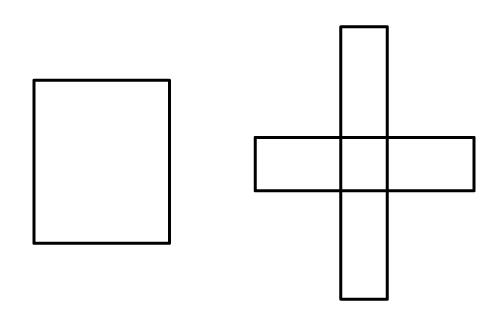Solution:

(i) It is given that length of each side of the slab = ½ m

One side of the square is formed by three slabs in a square arrangement

Length of side = 3 × ½ = 3/2 m

So the perimeter of the square arrangement = 4 × 3/2 = 6 m

(ii) From the figure, cross arrangement has 8 sides which form periphery of the arrangement and measure 1 m each.

It also has 4 sides which measure ½ m each

Perimeter of the cross arrangement = 1 + ½ + 1 + 1 + ½ + 1 + 1 + ½ + 1 + 1 + ½ + 1 = 8 + 2 = 10 m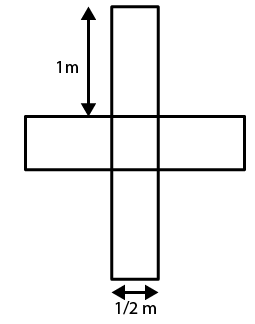(iii) We know that

Perimeter of cross arrangement = 10 m

Perimeter of square arrangement = 6 m

Hence, the perimeter of cross arrangement is greater than the perimeter of square arrangement.

(iv) No, Avneet cannot arrange the slabs having perimeter more than 10 m.